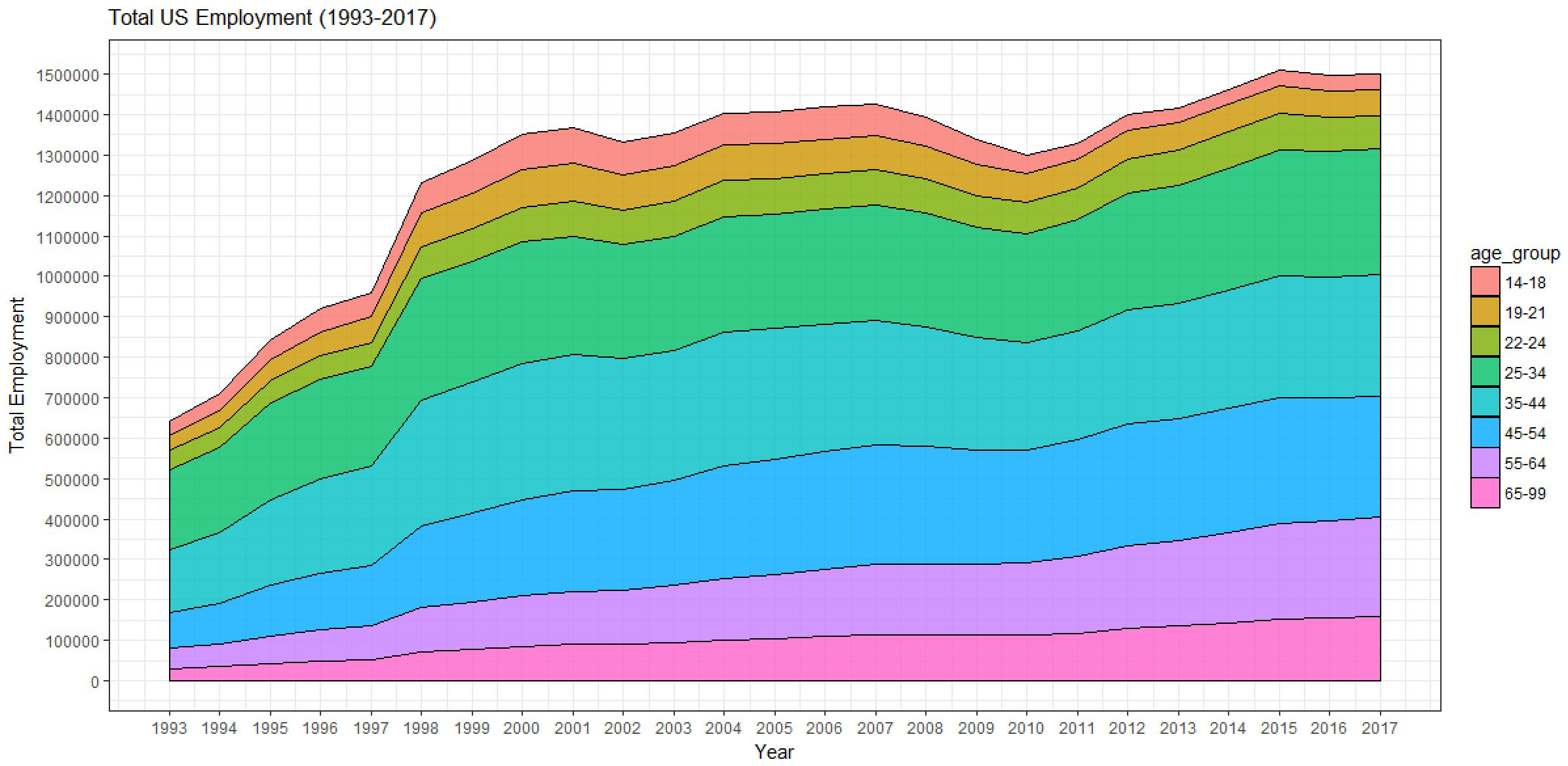Want to share your content on R-bloggers? click here if you have a blog, or here if you don't.

Before I can make a chart, I need to have some data. For this example, I will load a CSV data set which contains the total number of agriculture jobs by age group in the US from 1993 to 2017.  I add a data frame containing this data by using the read.csv()function, and then preview the data set by using the head()function.

```df = read.csv("employment.csv", header = TRUE)
```

We’ll now use this data frame to create the area chart using the ggplot2 package.

## Creating an area chart

First, I’ll load the ggplot2 library in R.

```library(ggplot2)
```

Next, I call the ggplot() function to create the plot object.

```plot = ggplot(df, aes(x=year, y=employment, fill=age_group))
```

This specifies the data frame to be input to the ggplot function, and it defines which variables will be used as the x-axis, y-axis and fill values. In this case, I want the plot the year on the x-axis with the total employment count on the y-axis. The fill parameter is optional, and if it is omitted a single value area chart will be created. Here I have instead specified the age_group variable, and this will result in a stacked area chart by age group.

Next, I call the geom_area() function to draw the area chart.

```plot + geom_area()
```

The above chart was created with the default ggplot2 settings, but there are several chart formatting options that can be modified.  In ggplot2 you can build a plot in stages. This means that you can sequence the functions for modifying the plot by “adding” them with a “+” sign. This is used to separate the different function calls.  The code below applies a series of additional formatting functions to the chart above. I have broken up these functions across multiple lines to help with readability, but these can all be on one line as well.

```plot +
geom_area(colour="black", size=.2, alpha=.8) +
theme_bw() +
scale_x_continuous(breaks=seq(1993,2017,1)) +
scale_y_continuous(breaks=seq(0,2000000,100000)) +
ggtitle("Total US Employment (1993-2017)") +
labs(x="Year", y="Total Employment")
```The code above builds this area chart by:

• Starting with a default area chart.
• Adding standard black/white themed background colors.
• Adding x-axis labels and scalings.
• Adding y-axis labels and scalings.
• Adding x-axis and y-axis labels.

There are a wide range of additional properties that can be modified in the ggplot2 package including chart and axis titles, borders, grid lines, legend, etc. A complete list of properties and attributes can be found on the the ggplot2 webpage.

To discover more about all the things you can do in R, check out our “R” guides